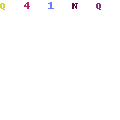# Lace Front Wigs Stock List

 `100% indian virgin hair` `Length  (in)` `Color` `texture` `Quantity (pcs)` `8”` `#1` `natural straight` ` 18` `10”` `#1` `natural straight` ` 15` `12”` `#1` `natural straight` ` 17` `14”` `#1` `natural straight` ` 38` `16”` `#1` `natural straight` ` 15` `18”` `#1` `natural straight` ` 22` `20”` `#1` `natural straight` ` 48` `22”` `#1` `natural straight` ` 34` `24”` `#1` `natural straight` ` 21` `8”` `#1b` `natural straight` ` 29` `10”` `#1b` `natural straight` ` 28` `12”` `#1b` `natural straight` ` 36` `14”` `#1b` `natural straight` ` 26` `16”` `#1b` `natural straight` ` 15` `18”` `#1b` `natural straight` ` 25` `20”` `#1b` `natural straight` ` 36` `22”` `#1b` `natural straight` ` 20` `24”` `#1b` `natural straight` ` 11` `8”` `#2` `natural straight` ` 14` `10”` `#2` `natural straight` ` 30` `12”` `#2` `natural straight` ` 31` `14”` `#2` `natural straight` ` 20` `16”` `#2` `natural straight` ` 49` `18”` `#2` `natural straight` ` 41` `20”` `#2` `natural straight` ` 45` `22”` `#2` `natural straight` ` 21` `24”` `#2` `natural straight` ` 14` `8"` `#4` `natural straight` ` 8` `10”` `#4` `natural straight` ` 20` `12”` `#4` `natural straight` ` 20` `14”` `#4` `natural straight` ` 15` `16”` `#4` `natural straight` ` 38` `18”` `#4` `natural straight` ` 46` `20”` `#4` `natural straight` ` 40` `22”` `#4` `natural straight` ` 20` `24”` `#4` `natural straight` ` 13` `8”` `#1` `yaki straight` ` 8` `10”` `#1` `yaki straight` ` 7` `12”` `#1` `yaki straight` ` 28` `14”` `#1` `yaki straight` ` 25` `16”` `#1` `yaki straight` ` 20` `18”` `#1` `yaki straight` ` 50` `20”` `#1` `yaki straight` ` 12` `22”` `#1` `yaki straight` ` 17` `8”` `#1b` `yaki straight` ` 9` `10”` `#1b` `yaki straight` ` 25` `12”` `#1b` `yaki straight` ` 32` `14”` `#1b` `yaki straight` ` 48` `16”` `#1b` `yaki straight` ` 30` `18”` `#1b` `yaki straight` ` 48` `20”` `#1b` `yaki straight` ` 38` `22”` `#1b` `yaki straight` ` 28` `8"` `#2` `yaki straight` ` 4` `10”` `#2` `yaki straight` ` 7` `12”` `#2` `yaki straight` ` 17` `14”` `#2` `yaki straight` ` 30` `16”` `#2` `yaki straight` ` 20` `18”` `#2` `yaki straight` ` 28` `20”` `#2` `yaki straight` ` 16` `22”` `#2` `yaki straight` ` 19` `8”` `#4`, `yaki straight` ` 11` `10”` `#4` `yaki straight` ` 11` `12”` `#4` `yaki straight` ` 30` `14”` `#4` `yaki straight` ` 37` `16”` `#4` `yaki straight` ` 27` `18”` `#4` `yaki straight` ` 18` `20”` `#4` `yaki straight` ` 33` `22”` `#4` `yaki straight` ` 14` ` 24"` `#4` `yaki straight` `  2` ` ` ` ` ` ` ` ` ` ` ` `
SMALL OR MEDIUM SIZE ARE AVAILABLE.
``